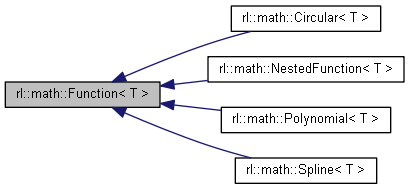Robotics Library  0.7.0
rl::math::Function< T > Class Template Referenceabstract

A mathematical mapping from Real -> ArrayX. More...

#include <Function.h>

Inheritance diagram for rl::math::Function< T >:[legend]

## Public Member Functions

Function ()

virtual ~Function ()

virtual Functionclone () const =0

Real duration () const

Reallower ()

const Reallower () const

Realupper ()

const Realupper () const

virtual T operator() (const Real &x, const ::std::size_t &derivative=0) const =0
Evaluates the function or a derivative thereof for a given value x. More...

Real x0

Real x1

## Detailed Description

### template<typename T> class rl::math::Function< T >

A mathematical mapping from Real -> ArrayX.

A Function is guaranteed to be defined in the interval [lower() upper()], and may be defined outside this interval. Its computation is expected to be numerically stable, accurate and efficient.

## ◆ Function()

template<typename T >
 rl::math::Function< T >::Function ( )
inline

## ◆ ~Function()

template<typename T >
 virtual rl::math::Function< T >::~Function ( )
inlinevirtual

## ◆ clone()

template<typename T >
 virtual Function* rl::math::Function< T >::clone ( ) const
pure virtual

## ◆ duration()

template<typename T >
 Real rl::math::Function< T >::duration ( ) const
inline

## ◆ lower() [1/2]

template<typename T >
 Real& rl::math::Function< T >::lower ( )
inline

## ◆ lower() [2/2]

template<typename T >
 const Real& rl::math::Function< T >::lower ( ) const
inline

## ◆ operator()()

template<typename T >
 virtual T rl::math::Function< T >::operator() ( const Real & x, const ::std::size_t & derivative = 0 ) const
pure virtual

Evaluates the function or a derivative thereof for a given value x.

Some functions are only defined in the interval [lower(), upper()], and fail to evaluate outside of [lower() - FUNCTION_BOUNDARY, upper() + FUNCTION_BOUNDARY]. In Debug mode, this is signaled by failing asserts. In Release mode, the function is evaluated if algebraically possible, or will return an empty ArrayX otherwise. Some functions are not indefinitely often differentiable, and will return a NaN array for all higher orders.

Parameters
 [in] x Input value of the function or derivative [in] derivative Order of the derivative to be evaluated

## ◆ upper() [1/2]

template<typename T >
 Real& rl::math::Function< T >::upper ( )
inline

## ◆ upper() [2/2]

template<typename T >
 const Real& rl::math::Function< T >::upper ( ) const
inline

## ◆ x0

template<typename T >
 Real rl::math::Function< T >::x0
protected

## ◆ x1

template<typename T >
 Real rl::math::Function< T >::x1
protected

The documentation for this class was generated from the following file: